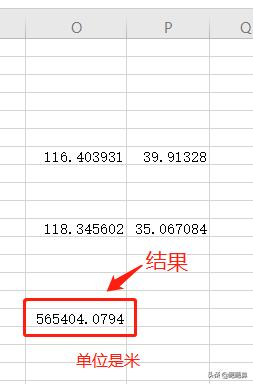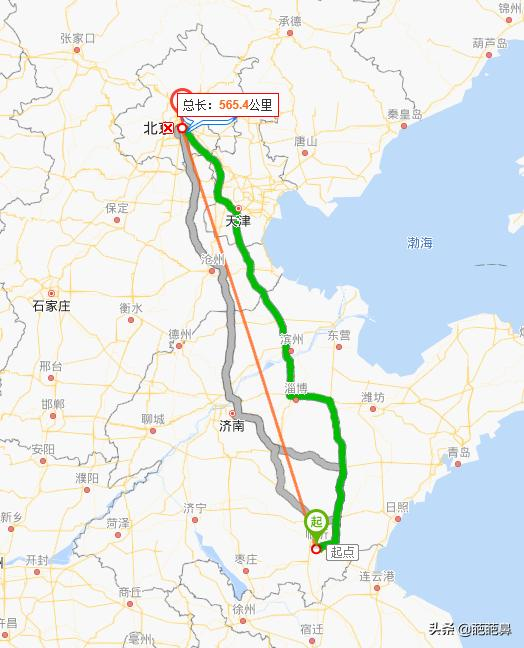# 公式计算软件（两点坐标距离公式计算软件）O列的经度，P列为纬度

=6371004*ACOS(1-(POWER((SIN((90-P7)*PI()/180)*COS(O7*PI()/180)-SIN((90-P11)*PI()/180)*COS(O11*PI()/180)),2)+POWER((SIN((90-P7)*PI()/180)*SIN(O7*PI()/180)-SIN((90-P11)*PI()/180)*SIN(O11*PI()/180)),2)+POWER((COS((90-P7)*PI()/180)-COS((90-P11)*PI()/180)),2))/2)